# Integral of sec²(x)/exp(tan(x))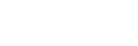Although it may look a bit intimidating at first, I can guarantee you this is actually a very easy integral!

But what integration technique do you think will be useful? You might think we could perform integration by parts, but I really don’t think it’s a good idea to find the integral of sec²(x) or 1/exp(tan(x)). What we need is u-substitution. The “hard” part is to choose what to substitute. If you remember, the derivative of tan(x) is sec²(x), which we have at the numerator, and all we have to do is simply substitute sec²(x)dx with du and we will be left with 1/exp(u), which is way better!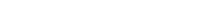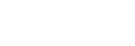Since sec²(x)dx is du we will write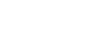Let’s now talk about the function exp: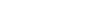and, in general,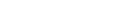This means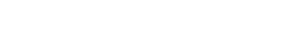We know that the integral of e^u is equal to e^u, so this integral is –e^-u (simply apply the chain rule); therefore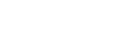At this point we can undo the substitution (remember that u=tan(x)):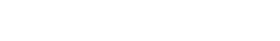Overall: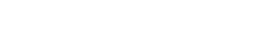That’s it! I really hope you liked this post and if so, give it a like! If you want to stay updated subscribe, it would mean a lot to me! Thanks!

Join 31 other subscribers.